Games
Problems
Go Pro!

# Math

Pro Problems > Math

# Featured Pro Problems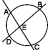Complex numbers, sequences and series, trigonometry, coordinate geometry, and conics all come into play in this hodge-podge problem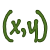Find the sum of x and y, if the following quadratic equations in x and y are true.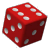Calculate the sum of squares, given the arithmetic, geometric and harmonic means of three numbersThree digits get together for brunch and discuss their relationships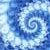Without a calculator find a nine digit number that meets the criteria of the problemA math problem involving Shrek wanting Fiona's telephone numberThe digits from zero to nine are competitors in a new reality TV show. Which digit will win?Two people go to the same store and make the same purchases, but one of them is treated unfairly in the pricing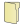# Full Directory Listing

Series of CirclesLike us on Facebook to get updates about new resources